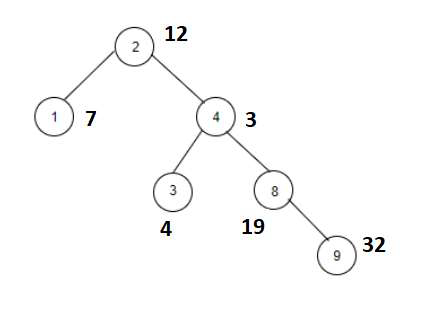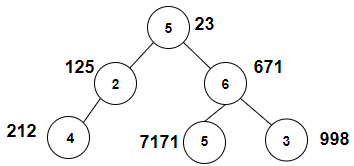# Count the nodes whose sum with X is a Fibonacci number in C++

Given a binary tree with weights of its nodes as numbers. The goal is to find the number of nodes that have weights such that the number is a Fibonacci number. Numbers in Fibonacci series are: 0, 1, 1, 2, 3, 5, 8, 13….nth number is the sum of (n−1)th and (n−2)th. If weight is 13 then it is a Fibonacci number so the node will be counted.

For Example

## Input

temp =1. The tree which will be created after inputting the values is given below −## Output

Count the nodes whose sum with X is a Fibonacci number are: 3

## Explanation

we are given with the tree nodes and the weights associated with each
node. Now we check whether the temp+weight is a Fibonacci number or not.

NodeWeightWeight+temp=fibonacciYes/No
21212+1=13yes
177+1=8yes
433+1=4no
344+1=5yes
81919+1=20no
93232+1=33no

## Input

temp = 3. The tree which will be created after inputting the values is given below −## Output

Count the nodes whose sum with X is a Fibonacci number are: 3

## Explanation

we are given with the tree nodes and the weights associated with each
node. Now we check whether the temp+weight is a Fibonacci number or not.

NodeWeightWeight+temp=fibonacciYes/No
52323+3=26no
2125125+3=128no
6671671+3=674no
4212212+3=215no
571717171+3=7174no
3998998+3=1001no

Approach used in the below program is as follows

In this approach we will apply DFS on the graph of the tree to traverse it and check if the weights of the node and temp add upto a fibonacci number. Take two vectors Node_Weight(100) and edge_graph for this purpose.

• Initialize Node_Weight[] with the weights of nodes.

• Create a tree using vector edge_graph.

• Take a global variable Fibonacci and initialize it with 0. Take other global variable temp.

• Function check_square(long double val) takes an integer and returns true if val is a perfect square.

• Take val_1 = sqrt(val)

• Now if(val_1 − floor(val_1) == 0) returns true then total is a perfect square, return true.

• Return false otherwise.

• Function check_Fibonacci(int num) takes a number and returns true if it is a fibonacci number.

• Initialize fib with 5*num*num.

• If check_square((fib + 4)) || check_square((fib − 4)) results true then return true.

• Return false otherwise.

• Function Fibonacci_number(int node, int root) returns count of nodes whose sum with X is a Fibonacci number.

• If if(check_Fibonacci(Node_Weight[node] + temp)) returns true then increment Fibonacci.

• Traverse tree in vector edge_graph[node] using for loop.

• Call Fibonacci_number(it, node) for the next node in the vector.

• At the end of all functions we will have a Fibonacci as the number of nodes with weights having sum with temp as a fibonacci number.

## Example

Live Demo

#include <bits/stdc++.h>
using namespace std;
vector<int> Node_Weight(100);
vector<int> edge_graph;
int Fibonacci = 0, temp;
bool check_square(long double val){
long double val_1 = sqrt(val);
if(val_1 − floor(val_1) == 0){
return true;
}
return false;
}
bool check_Fibonacci(int num){
int fib = 5 * num * num;
if(check_square((fib + 4)) || check_square((fib − 4))){
return true;
}
return false;
}
void Fibonacci_number(int node, int root){
if(check_Fibonacci(Node_Weight[node] + temp)){
Fibonacci++;
}
for (int it : edge_graph[node]){
if(it == root){
continue;
}
Fibonacci_number(it, node);
}
}
int main(){
//weight of the nodes
Node_Weight = 6;
Node_Weight = 4;
Node_Weight = 23;
Node_Weight = 5;
Node_Weight = 161;
Node_Weight = 434;
//create graph edge
edge_graph.push_back(1);
edge_graph.push_back(4);
edge_graph.push_back(3);
edge_graph.push_back(8);
edge_graph.push_back(9);
temp = 3;
Fibonacci_number(2, 2);
cout<<"Count the nodes whose sum with X is a Fibonacci number are: "<<Fibonacci;
return 0;
}

## Output

If we run the above code it will generate the following output −

Count the nodes whose sum with X is a Fibonacci number are: 1